COMPLEX NUMBERS

(notes by Roberto Bigoni)

1. Rectangular and polar plane coordinates.

Each point P of a Euclidean plane, if we define in this plane an orthogonal and monometric frame of reference Oxy, can be put in correspondence with an ordered pair of real numbers which are said its coordinates.

We can do this in two ways:

1. we draw from P the straight line perpendicular to the x-axis that intersects the axis in the point X; the distance OX, positive if X is on the right of O, negative if X in on the left or null if X coincides with O, is called the abscissa of P; in an analogous way, the straight line perpendicular to the y-axis gives the ordinate of P.

Abscissa and ordinate, commonly represented by x and y, are the cartesian (or rectangular) coordinates of the point P.

2. we draw the segment PO; the length of this segment (always non-negative) is the radius of P, commonly represented by ρ; the angle XOP (positive it counterclockwise, otherwise negative or null, commonly represented by θ) is the polar angle of P.

Here and below we assume -π < θπ, but in general we could take any value θ + 2kπ, k ∈ Z.

Radius and polar angle are the polar coordinates of P.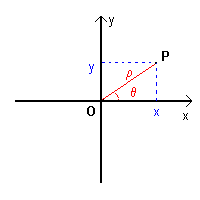Rectangular and polar coordinates are linked by the following identities: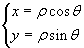Vice versa we have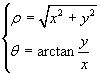Since the tangent function is bijective only in the interval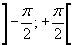, the second one of these equalities is immediately true if P lies in the first or fourth quadrant; if P lies in the second quadrant, to get its polar angle we must add π to the arctangent; if P lies in the third quadrant, to get its polar angle we must subtract π from the arctangent.

The following Javascript application translates the coordinates from rectangular to polar.
Obviously it may work only if your browser allows the execution of scripts.
If your browser does not allow internal frames, you can directly access the application page.

Given the bijectivity between points of a plan and pairs of real number, hereafter the word point will be synonimous of pair of reals, without reference to its geometrical meaning.

2. Sum of points.

Given the rectangular coordinates of two points P and Q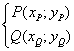we can obtain the rectangular coordinates of a third point S in the following way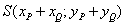One can legitimately say that S is the sum of P and Q because the relationship between P, Q and S has all the formal properties of the sum of two real numbers α, β and their sum σ = α + β.

In fact, it is internal, associative and commutative, admits a neutral element and each element is invertible.

The set of the points together with this sum is an algebraic structure. More precisely, it is an abelian group.

In this group, the neutral element Z, that is the point that combined with any other point P(xP;yP) leaves it unchanged, expressed by rectangular coordinates, is Z(0;0). The radius of Z is 0, its polar angle is undetermined.

The additive inverse of P(xP;yP) (or its opposite), that is the point that combined with P gives Z, is -P(-xP;-yP).

Moreover, we can define a multiple of order n of any point P as the sum of n (nN) identical points P, and, more generally, a multiple of order α (α ∈ R):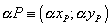3. Product of points.

If we now focus our attention on the polar coordinates of two points P and Q: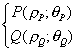we can obtain the polar coordinates of a third point T in the following way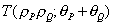that is the point T whose radius ρT is the the product of ρP and ρQ and whose polar angle is the sum of θP and θQ. If this sum is out from the range ]-π;π] we'll normalize it by adding or subtracting how many 2π are needed.

We can say that T is the product of P and Q because the relationship between P, Q and T has all the formal properties of the product of two real numbers.

In fact, also this relationship is internal, associative and commutative, admits a neutral element and each element is invertible.

The neutral element U has radius 1 and polar angle 0. Every point P(ρ ; θ) has its multiplicative inverse (or reciprocal)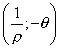Obviously the point Z, whose radius is 0, doesn't have the reciprocal and its product by any other point gives always Z: Z is the absorbent element.

4. The field of the complex numbers.

The set of the plane points C (i.e. the pairs of reals), together with both the operations of sum and product defined in sections 2 and 3, has the same algebraic structure as the set of the real numbers together with the common addition and multiplication; so it also is a field

.

This field, only due to historical reasons, is said the field of the complex numbers, because its elements (that is the pairs of reals numbers) are said complex numbers.

Each complex number can be represented either by its rectangular coordinates or by its polar coordinates. The two representations can be used interchangeably.

Hereafter we'll call rectangular complex a complex number expressed by its rectangular coordinates and polar complex a complex number expressed by its polar coordinates.

The radius of a polar complex is usually called its modulus or its absolute value; the polar angle is usually called its argument.

If we represent by u and i the rectangular complexes u(1;0) and i(0;1), that is the unit vector of the x-axis and the unit vector of the y-axis, each rectangular complex z can be represented as a linear combination of u and i: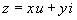Usually the unit vector u is omitted while i, said imaginary unit, must be expressed explicitly. So a rectangular complex z is written in the following way, said algebraic form of complex numbers: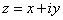In electronics, the imaginary unit is represented by the symbol j, since i is usually the intensity of electricity.

The coordinates x and y of a rectangular complex are called respectively real part and imaginary part.

When the real part is 0, it is omitted. So we write, for example,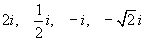.

Also when the imaginary part is 0 it is omitted. So we write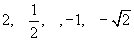. But these aren't real numbers. They are simplified expressions of the complex numbers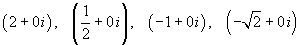.

To better explain: if -1 is a real number, we can't calculate its square root, because there is no real number which has a negative square. But (-1 + 0 i) is a complex number and we'll see that there are complex numbers whose square is (-1 + 0 i), so we can calculate its square root.

The set of the complex numbers with imaginary part equal to 0, represented in a cartesian plane, coincides with the x-axis which, in this context, is said the real axis, while the set of the complex numbers with real part equal to 0, represented in a cartesian plane, coincides with the y-axis which is said the imaginary axis. Using these two axes we can plot each complex number as a point of a plane called complex plane.

The polar coordinates of the imaginary unit i are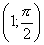The polar coordinates of the square of i are (1 ; π). The rectangular coordinates of this square are (-1 ; 0).

So we can write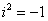(Remember that -1 is a complex number).

The polar complex z(ρ ; θ) is the same as the rectangular complex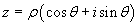This expression is called trigonometric form of complex numbers.

Using the Euler's formulas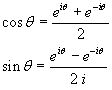we obtain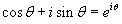and we can also write a polar complex in the following way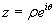This expression is called exponential form of complex numbers.

Usually complex numbers, depending on the context, or are written in algebraic form, referring to their rectangular coordinates, or in trigonometric form and, preferably, in exponential form, referring to their polar coordinates.

Example:

The rectangular complex z(1 ; 1) has the algebraic form 1 + i.

To obtain its reciprocal we can express z in trigonometrical form: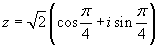The reciprocal of z in trigonometric form is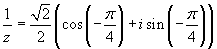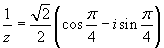The reciprocal of z in exponential form is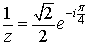The reciprocal of z in algebraic form is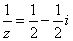It is also possible to determine the reciprocal directly from the algebraic form.

For this purpose it is convenient to define for each rectangular complex z = x + i y the rectangular complex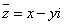that is the rectangular complex we can obtain from z changing the sign of its imaginary part; this number is called the conjugate of z.

The product of a complex number by its conjugate coincides with ρ2, that is the square of its modulus: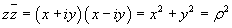To obtain directly the reciprocal of a rectangular complex we observe that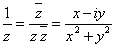The reciprocal of a complex number is equal to the ratio of its conjugate and the square of its modulus.

In the example shown above we have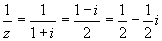5. Inverse operations on complex numbers.

As in all algebraic fields, also in the complex field:

• the subtraction is the sum of the minuend with the opposite of subtrahend;
• the division is the product of the dividend by the reciprocal of the divisor.

The subtraction is immediate between rectangular complexes, while the division is immediate between polar complexes but remains fairly simple even between rectangular complexes.

Example.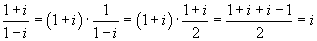6. Integer power and integer roots of the complex numbers (De Moivre's formula).

Like in the field of the real numbers, the n-th power (n ≥ 2) of a number z is given by the product of n factors equal to z. Given the polar number z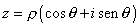we have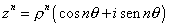Using the exponential form we have more directly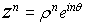We note that, given -π < θ ≤ π, the argument of the n-th power may be out from this range; in this case, as we have seen for the product, we'll normalize it by adding or subtracting how many 2π are needed.

Vice versa, a n-th root of z is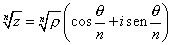because its n-th power is z.

We'll call this root the principal n-th root of z.

But since sine and cosine have period 2π, every argument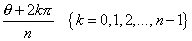is the argument of a n-th root, because sines and cosines of each of these arguments multiplied by n are identical.

So, in general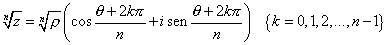o, in exponential form,Many textbooks begin their discussion of complex numbers by saying that i is the square root of -1. This statement is incorrect if -1 is given as real number: real numbers don't have negative squares, so there aren't square roots of negative real numbers. But it is incorrect even if -1 is given as complex number, because complex numbers have always two square roots: the two square roots of the complex number -1 are +i and -i.
Moreover it is a grave mistake to say that complex numbers allow to extract the square root of negative numbers: the set of the complex number is not ordered so we can not say whether a complex number is greater or less than 0, that is complex numbers can not be classified as neither positive nor negative.

Example.

Given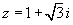, what are the fifth roots of z5?

The polar coordinates of z are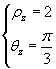so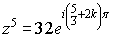from where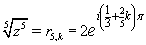In detail, the five roots are: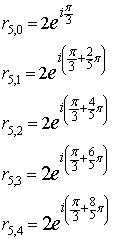if we want to write these roots in algebraic form, we have obviously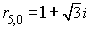This is the principal root.

To calculate the cosine and the sine of the arguments of the other four roots we remember that in a golden triangle of the first type the ratio between the base and the side is φ, so we have

•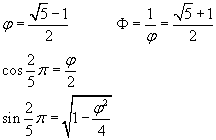•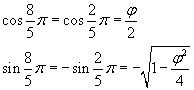•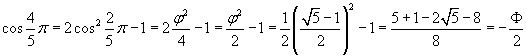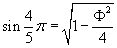•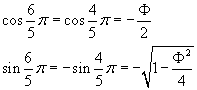Now we can use the addition formulas and obtain, for example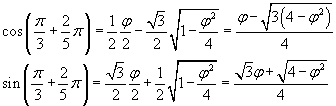an finally7. Complex number raised to complex exponent.

If we express the base in exponential form and the exponent in algebraic form, the power p with base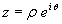and exponent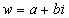can be written as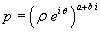.

By applying the known properties of the powers we get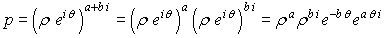The factors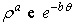are evidently real, while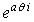is evidently a complex with modulus 1. To understand the nature of the factor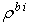we may observe that every power rx can be written as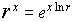so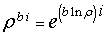.

Ultimately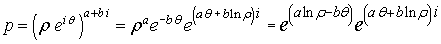Here the first exponential gives the modulus of the power while the real factor of the second exponent gives the argument of the power. If this argument is out from the range ]-π;π] we'll normalize it by adding or subtracting how many 2π are needed.

Examples.

•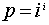We have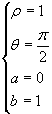Therefore: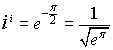•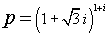We have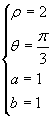Therefore:8. Logarithms of a complex number.

A logarithm of a complex number is immediate if the number is written in exponential form.

From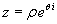we obtain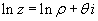In general the imaginary part of the logarithm could be any value, but here to simplify the discussion, we assume like above -π < θπ, that is we consider only the principal value of the logarithm.

Examples.

•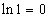•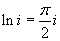•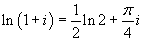9. Sine and cosine, circular and hyperbolic, of a complex number.

Given the rectangular complex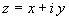, if we apply the addition formulas we obtain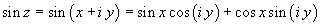From the Euler's formulas we have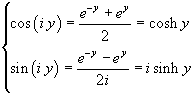so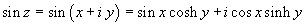Similarly we get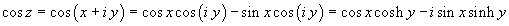With the hyperbolic functions we have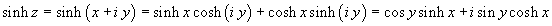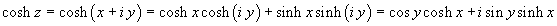Examples.

Given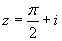•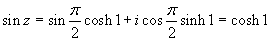•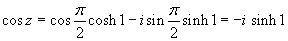•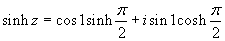•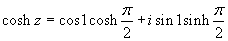test

In this site the page SuperCalculator allows you to perform calculations on complex numbers.

last revised: May 2018